Latest Banking jobs   »

# Quantitative Aptitude Quiz For IBPS Clerk/PO Prelims 2022- 30th August

Directions (1-5): In each of these questions, two equations (I) and (II) are given. You have to solve both the equations and give answer
(a) if x>y
(b) if x≥y
(c) if x<y
(d) if x ≤y
(e) if x = y or no relation can be established between x and y

Q1. I. 2x²-9x+9=0
II. 6y²-11y+4=0

Q2. I. x²-9x-10=0
II. y²-4y-5=0

Q3. I. x²+7x+10=0
II. y²-3y-4=0

Q4. I. x³=1728
II. y²+256=400

Q5. I. (x+5)³=343
II. (y-4)²=0

Directions (6-10): In each of the following questions two equations are given. Solve these equations and give answer:
(a) if x≥y, i.e., x is greater than or equal to y
(b) if x>y, i.e., x is greater than y
(c) if x≤y, i.e. x is less than or equal to y
(d) if x<y, i.e., x is less than y
(e) x=y or no relation can be established between x and y

Q6. I. x² -16x +55 = 0
II. y² + 16y + 55 = 0

Q7. I. x² – 20x + 75 = 0
II. y² -13y + 42 =0

Q8. I. 8x² -2x -45 = 0
II. y² -10y+21 =0

Q9. I. x² +8x + 15 = 0
II. y² +13y + 40 =0

Q10. I. x² – 9x +20 = 0
II. y²- 22y +85 = 0

Directions (11-15): In each of these questions, two equations (I) and (II) are given. You have to solve both the equations and give answer
(a) if x>y
(b) if x≥y
(c) if x<y
(d) if x ≤y
(e) if x = y or no relation can be established between x and y

Q11. I. 2x² – 9x + 9 = 0
II.6y² – 17y + 12 = 0

Q12. I.7y + 3x = 27
II.9y + 7x = 53

Q13. I.3x² + 5x – 112 = 0
II.2y² + 19y + 42 =0

Q14. I.28x² + 33x + 9 = 0
II.25y² + 50y + 24 = 0

Q15. I.x² – 39x + 360 = 0
II.y² – 49y + 600 = 0

Solutions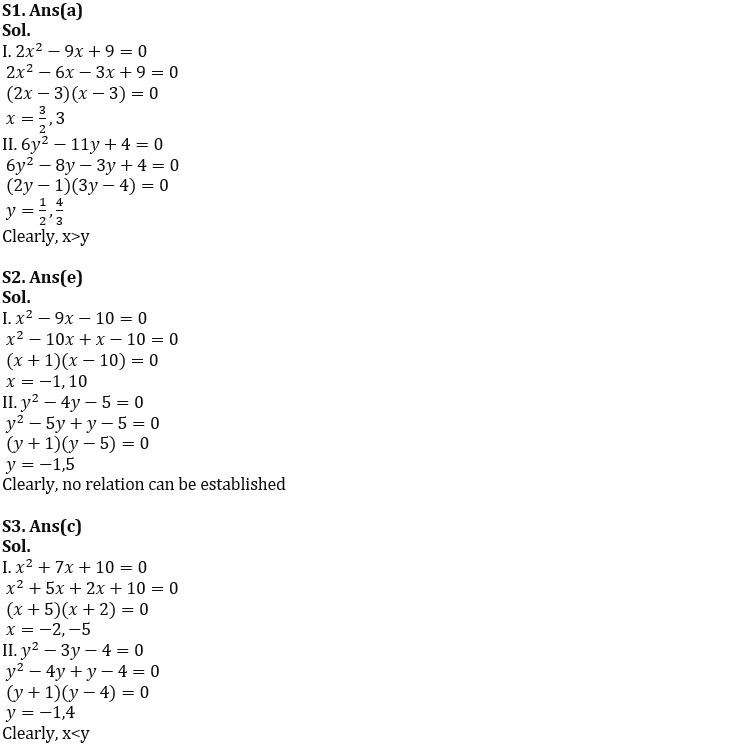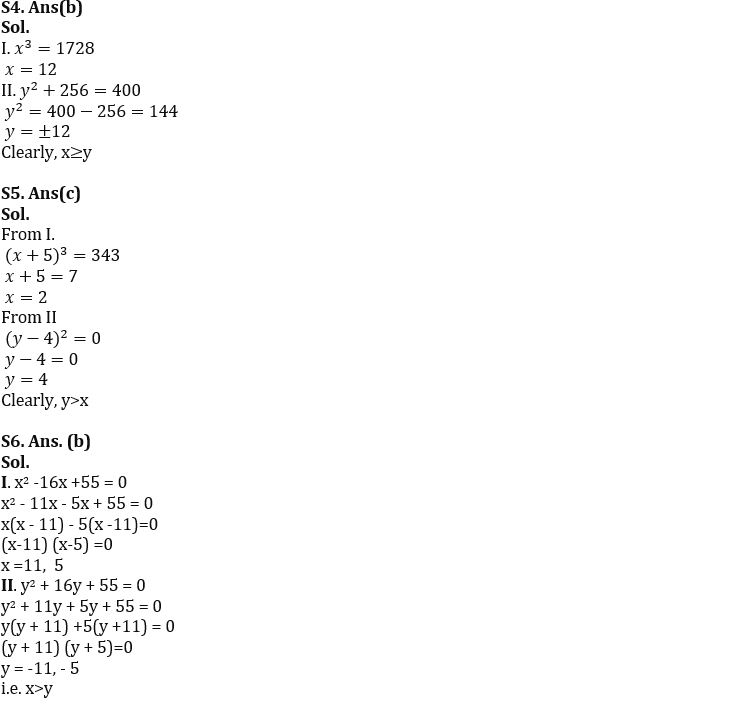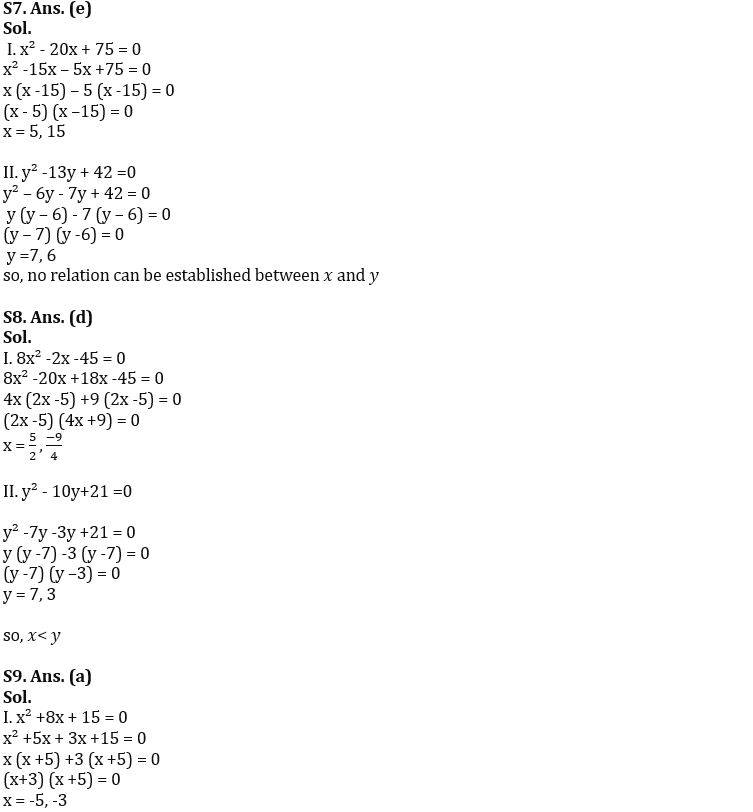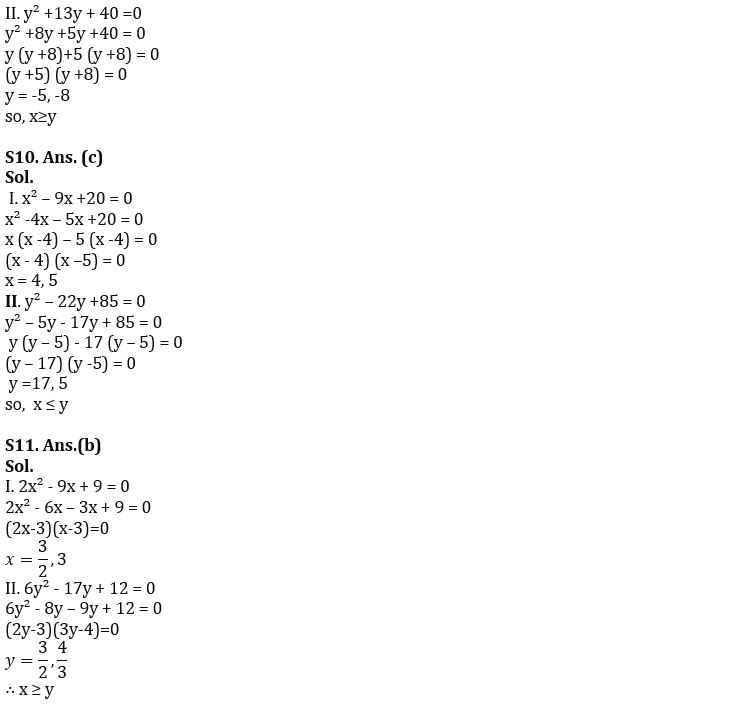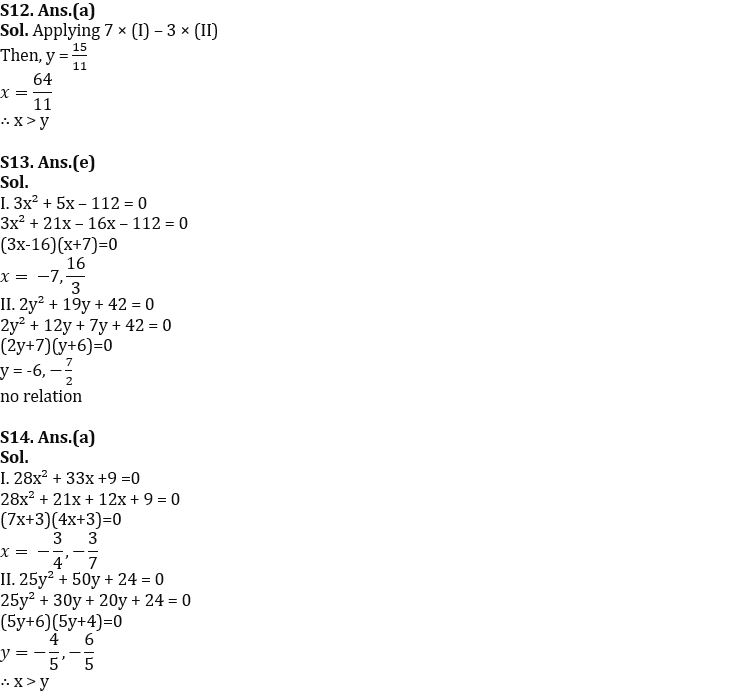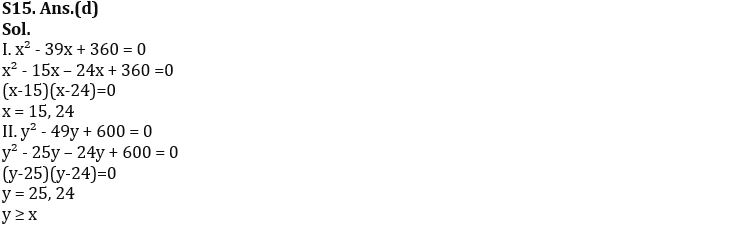#### Congratulations!Download Hindu Review of October 2021: Free PDF